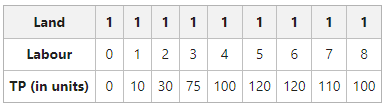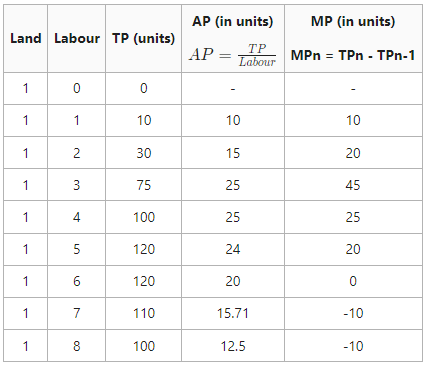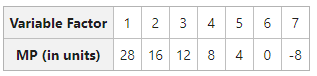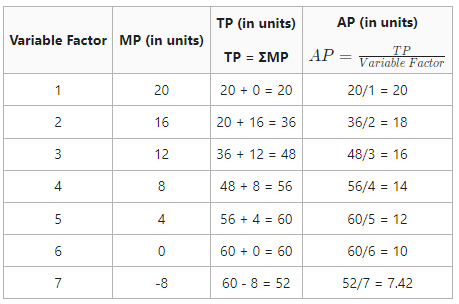# What is TP, AP and MP? Explain with examples.

Consumers and producers both play a crucial role in the efficient operation of an economy. A producer uses a variety of inputs to create commodities and services. Production is an essential economic activity since it transforms the product into the form consumers require, increasing its utility. It helps transform the raw materials into some desirable product. In simple words, it includes the transformation of input into output.

Production Function is an expression of the technological relationship between the physical inputs and outputs of a good.

The term “product” describes the volume of goods produced by a business or industry over a certain period.

The product concept can be seen from three different perspectives:

1. Total Product
2. Marginal Product
3. Average Product

### 1. Total Product (TP):

Total Product refers to the total quantity of goods produced by a firm during a given period of time with a given number of units. For example, if 5 labours produce 6 kg of wheat, then the total product is 30 kg. A company can increase TP in the short term by focusing primarily on the variable components. But over time, both fixed and variable elements can be increased to raise TP. Other names of Total Product are Total Physical Product, Total Return, or Total Output.

### 2. Average Product (AP):

Average Product refers to output per unit of a variable input. For example, if the total product is 30 kg of wheat produced by 5 labours(variable inputs), then the average product will be 30/5, i.e., 6 kg. AP is calculated by dividing TP by units of the variable factor.

Formula:TP in terms of AP will be:### 3. Marginal Product (MP):

Marginal Product refers to the addition to the total product when one more unit of a variable factor is employed. It calculates the extra output per additional unit of input while keeping all other inputs constant. Other names of Marginal Product are Marginal Physical Product (MPP) or Marginal Return.

Formula:

MPn = TPn – TPn-1

Where,

MPn = Marginal product of nth unit of the variable factor

TPn = Total product of n units of the variable factor

TPn-1 = Total product of (n-1) units of the variable factor

n = Number of units of the variable factor

For example, if 5 labours make 30 kg of wheat and 6 labours make 35 kg of wheat, then the MP of 6 labour will be:

MP6= TP6 – TP5

MP6= 35 – 30 = 5 kg

#### One more way to calculate MP

MP is the change in TP when one additional unit of variable factor is used. However, when the change in variable factor exceeds one unit, MP can be calculated as:For example, 2 labours produce 60 kg of rice and 4 labours produce 100 kg of rice, then MP will be:#### TP is summation of MP:

Total Product can also be calculated as the sum of marginal product.

It means,

TP = MP1 + MP2 + MP3 +…………………..MPn

or,

TP= ΣMP

#### Example 1:

Calculate AP and MP from the following particulars:#### Solution:#### Example 2:

Calculate TP and AP:#### Solution: